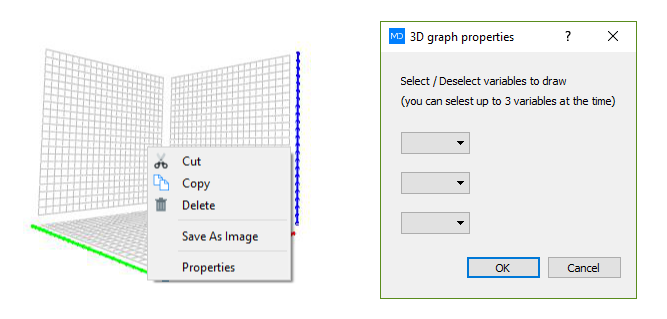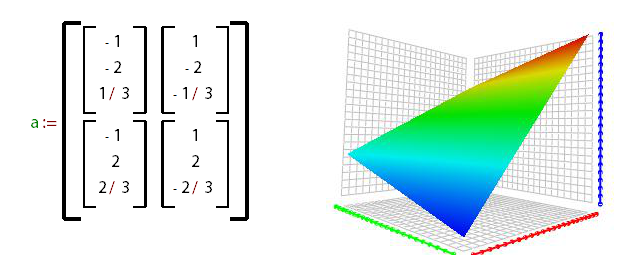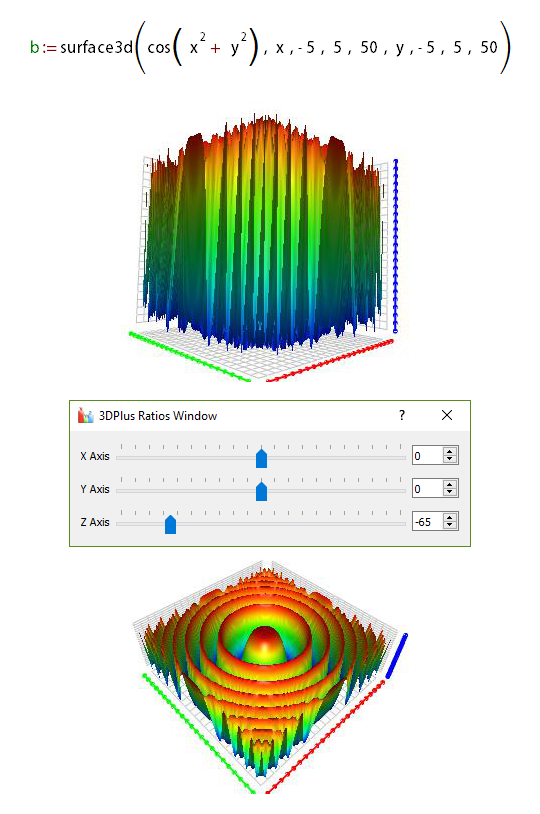# 3D Graph

To create 3D graphs in MatDeck you first have to define a variable with the matrix of vectors, where every vector represent a point in space. Therefore, vectors size is 3×1 and the minimal size of a matrix is 2×2. Graphs can only be placed inside the canvas.

First, define variable a:= and insert a matrix of vectors in it.

Choose Place tab→3D Graph icon, select a position on the canvas where you want to place your 3D graph and press the left mouse button. A graph object will appear. Press the right mouse button with your cursor hovering over the graph and select Properties. A new window will pop up.Choose variable a from the dropdown menu (only variable in our document). A new window, with graph in it, will open. Close it and you have drawn a 3D graph in your canvas.There are two functions that make up the matrix of vectors for the 3D graph. The functions are: surface3d – arguments are: function, first symbol, start value for the first symbol, end value for the first symbol, number of samples, second symbol, start value for the second symbol, end value for the second symbol, number of samples) , complexcurve2dre – arguments are: vector with two functions, starting value for the symbol, ending value for the symbol, number of samples.

Now we will use function sufrace3d to create a 3D graph. Type b:=surface3d to create a variable and a function in it. As your first argument, type cos to create a cosine function, and insert x2+y2 as its argument. Following arguments are x, -5, 5, 50, y, -5, 5, 50, meaning that intervals for x and y are [-5, 5], divided in 50 samples.

Choose the Place tab→3D Graph icon, select a position on the canvas where you would want to place a 3D graph and press the left mouse button. A graph object will appear. Press the right mouse button while your cursor is over the graph and go to Properties.

From the drop down menu choose the variable b. A new window will open, with graph in it. Click and drag on the graph to change the viewing angle. From the Coordinates tab choose the Ratios option and move Z Axis slider to -65. Close both windows and the last graph from underneath the canvas will appear.